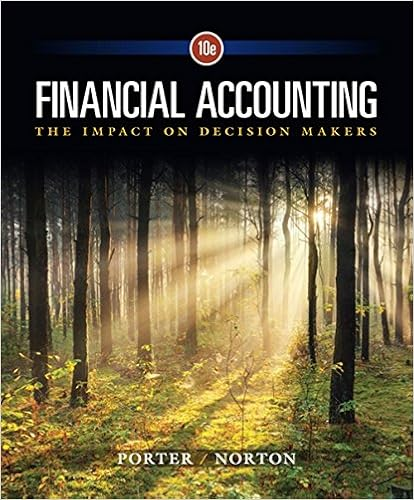# Your firm has a 300000 bond issue outstanding these

• Notes
• Haimeng
• 60
• 90% (139) 125 out of 139 people found this document helpful

This preview shows page 46 - 50 out of 60 pages.

##### We have textbook solutions for you!
The document you are viewing contains questions related to this textbook.The document you are viewing contains questions related to this textbook.
Chapter 12 / Exercise 12-19
Financial Accounting: The Impact on Decision Makers
Norton/PorterExpert Verified
57. Your firm has a \$300,000 bond issue outstanding. These bonds have a 7% coupon, pay interest semiannually, and have a current market price equal to 103% of face value. What is the amount of the annual interest tax shield given a tax rate of 35%? A. \$6,125 B. \$6,309 C. \$7,350 D. \$17,500 E. \$18,025 Annual interest tax shield = \$300,000 × .07 × .35 = \$7,350
Difficulty level: Easy Topic: Interest Tax Shield 58. Bertha's Boutique has 3,000 bonds outstanding with a face value of \$1,000 each and a coupon rate of 9%. The interest is paid semi-annually. What is the amount of the annual interest tax shield if the tax rate is 34%?
Difficulty level: Easy Topic: Interest Tax Shield 14-46
##### We have textbook solutions for you!
The document you are viewing contains questions related to this textbook.The document you are viewing contains questions related to this textbook.
Chapter 12 / Exercise 12-19
Financial Accounting: The Impact on Decision Makers
Norton/PorterExpert Verified
Chapter 14 - Capital Structure: Basic Concepts 59. Juanita's Steak House has \$14,000 of debt outstanding that is selling at par and has a coupon rate of 8%. The tax rate is 34%. What is the present value of the tax shield?
Difficulty level: Medium Topic: Interest Tax Shield 60. A firm has debt of \$5,000, equity of \$16,000, a leveraged value of \$8,900, a cost of debt of 8%, a cost of equity of 12%, and a tax rate of 34%. What is the firm's weighted average cost of capital?
Difficulty level: Medium Topic: Weighted Average Cost of Capital 14-47
Chapter 14 - Capital Structure: Basic Concepts 61. A firm has zero debt in its capital structure. Its overall cost of capital is 10%. The firm is considering a new capital structure with 60% debt. The interest rate on the debt would be 8%. Assuming there are no taxes or other imperfections, its cost of equity capital with the new capital structure would be: A. 9% B. 10% C. 13% D. 14% E. None of the above. rs = .11 + .60/.40(.11-.08) = .10 + .045 = .0145 = 14.5%
Difficulty level: Medium Topic: Cost of Equity 62. A firm has a debt-to-equity ratio of .60. Its cost of debt is 8%. Its overall cost of capital is 12%. What is its cost of equity if there are no taxes or other imperfections?
Difficulty level: Medium Topic: Cost of Equity 14-48
Chapter 14 - Capital Structure: Basic Concepts 63. A firm has a debt-to-equity ratio of 1. Its cost of equity is 16%, and its cost of debt is 8%. If there are no taxes or other imperfections, what would be its cost of equity if the debt-to- equity ratio were 0?
Difficulty level: Challenge Topic: Cost of Equity
•••